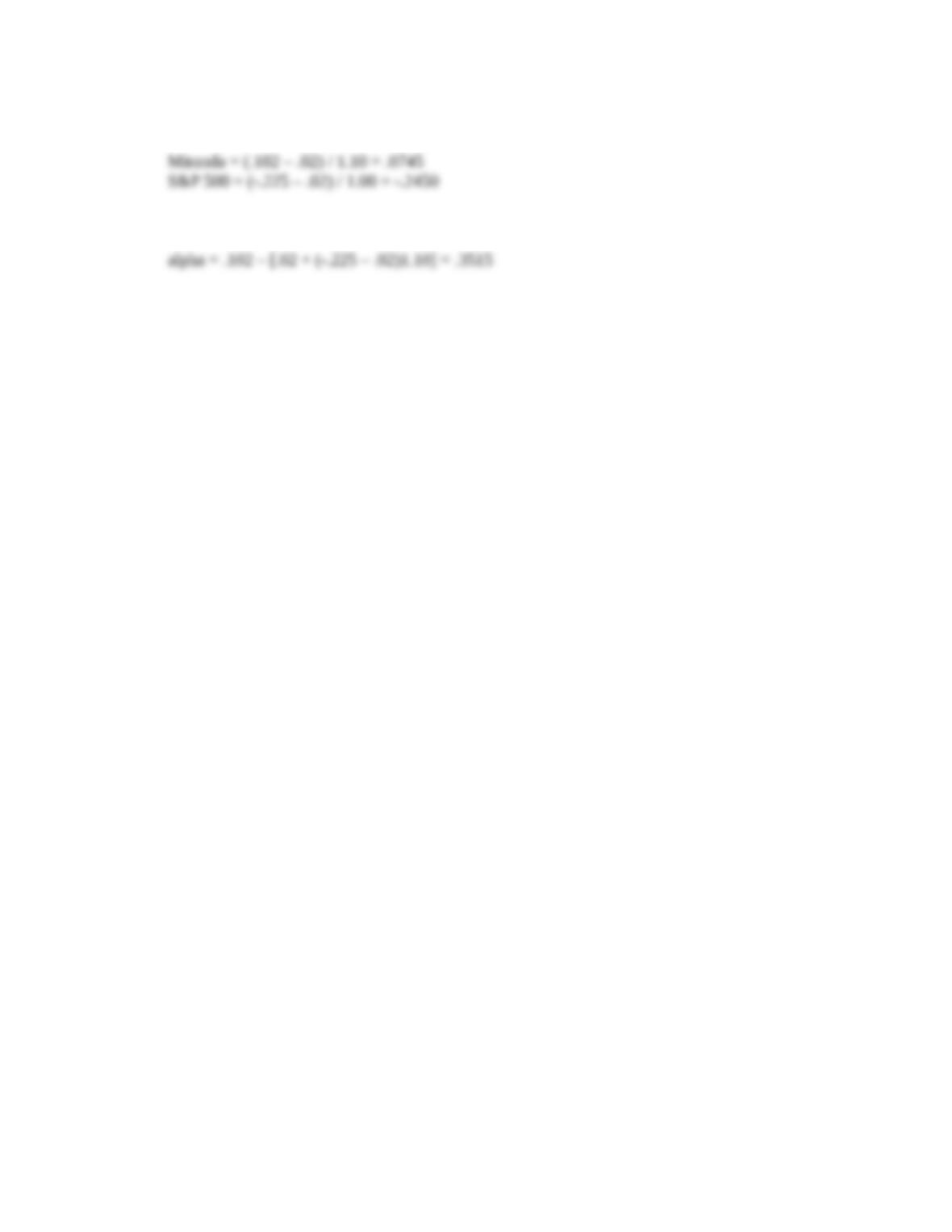Type
Solution Manual
Book Title
Fundamentals of Investments: Valuation and Management 8th Edition
ISBN 13
978-1259720697

### 978-1259720697 Chapter 13 Solution Manual

January 2, 2020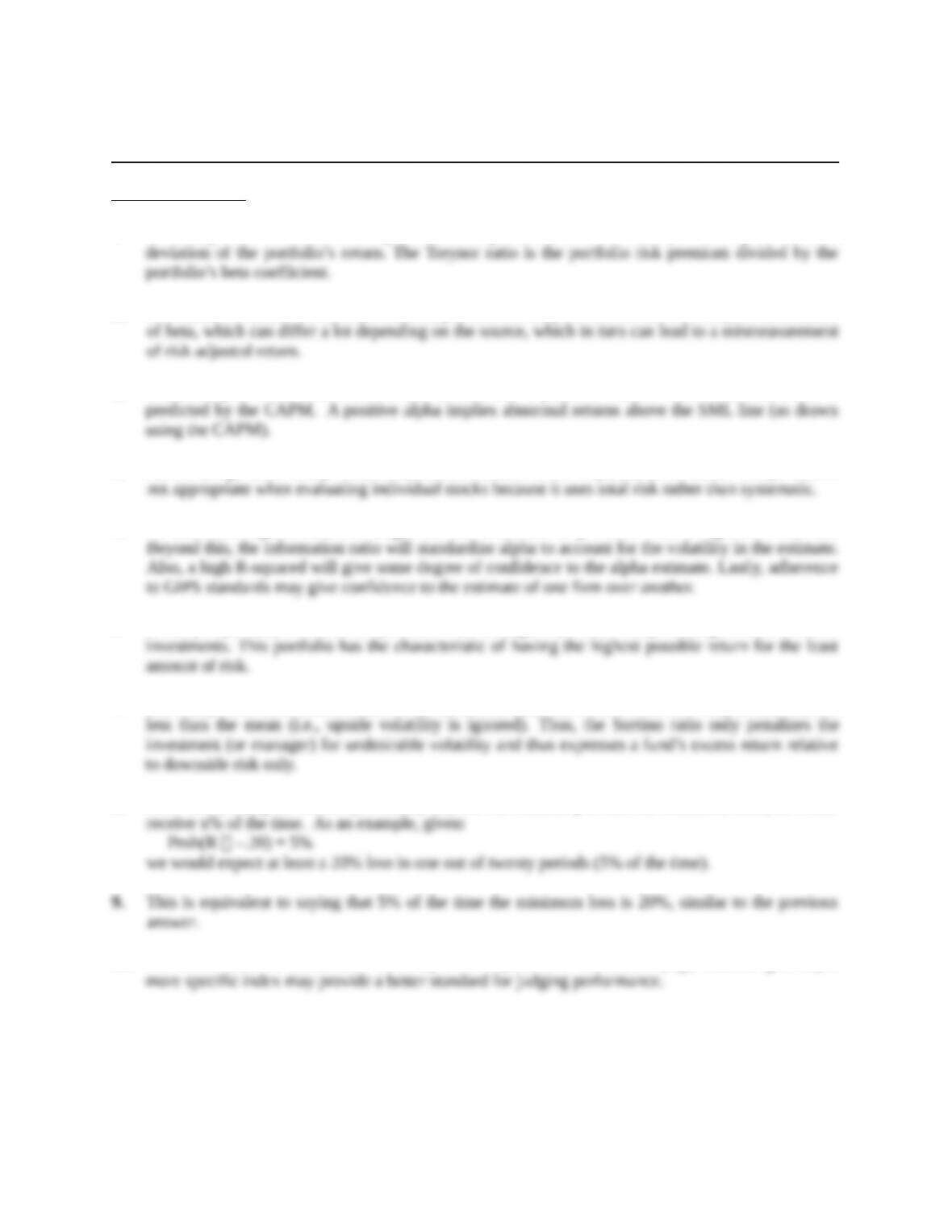Chapter 13
Performance Evaluation and Risk Management
Concept Questions
1. The Sharpe ratio is calculated as a portfolio’s risk premium, or excess return, divided by the standard
2. A common weakness of both the Jensen alpha and the Treynor ratio is that both require an estimate
3. Jensen’s alpha is the difference between a stock’s or a portfolio’s actual return and that which is
4. An advantage of the Sharpe ratio is that a beta estimate is not required; however, the Sharpe ratio is
5. To determine significance, one might use the t-statistics or p-values from a regression estimate.
6. A Sharpe optimal portfolio is the portfolio with the highest possible Sharpe ratio given the available
7. The Sharpe ratio uses total deviation from the mean, while the Sortino ratio uses only returns that are
8. After establishing the desired probability (x), the VaR statistic provides the minimum loss you would
10. For sector funds or investments that only cover a portion of the market (e.g., value or growth), a
Education.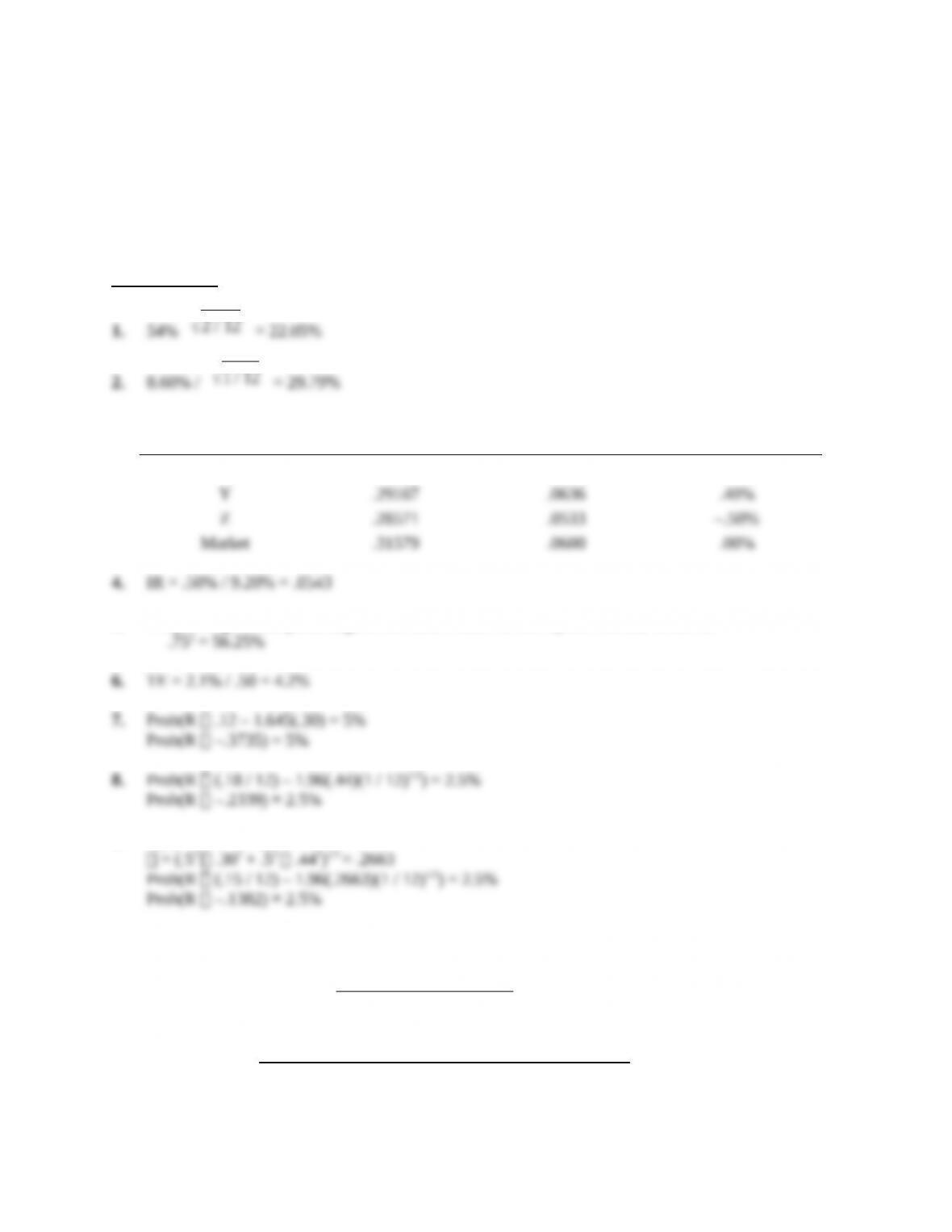Solutions to Questions and Problems
NOTE: All end of chapter problems were solved using a spreadsheet. Many problems require multiple
steps. Due to space and readability constraints, when these intermediate steps are included in this
solutions manual, rounding may appear to have occurred. However, the final answer for each problem is
found without rounding during any step in the problem.
Core Questions
3.
Portfolio Sharpe ratio Treynor ratio Jensen's alpha
X .27586 .0640 .50%
5. R-squared gives the percentage of the fund’s return driven by the market, which is:
9. E(R) = (.12 + .18) / 2 = .15
10. For a portfolio with two investments having zero correlation, the Sharpe ratio would be calculated as
follows:
2σB
2)1/2
11.
2+. 2(.5 )(.5 )(σS)(σB)(Corr (RS,RB))]1/2
Education.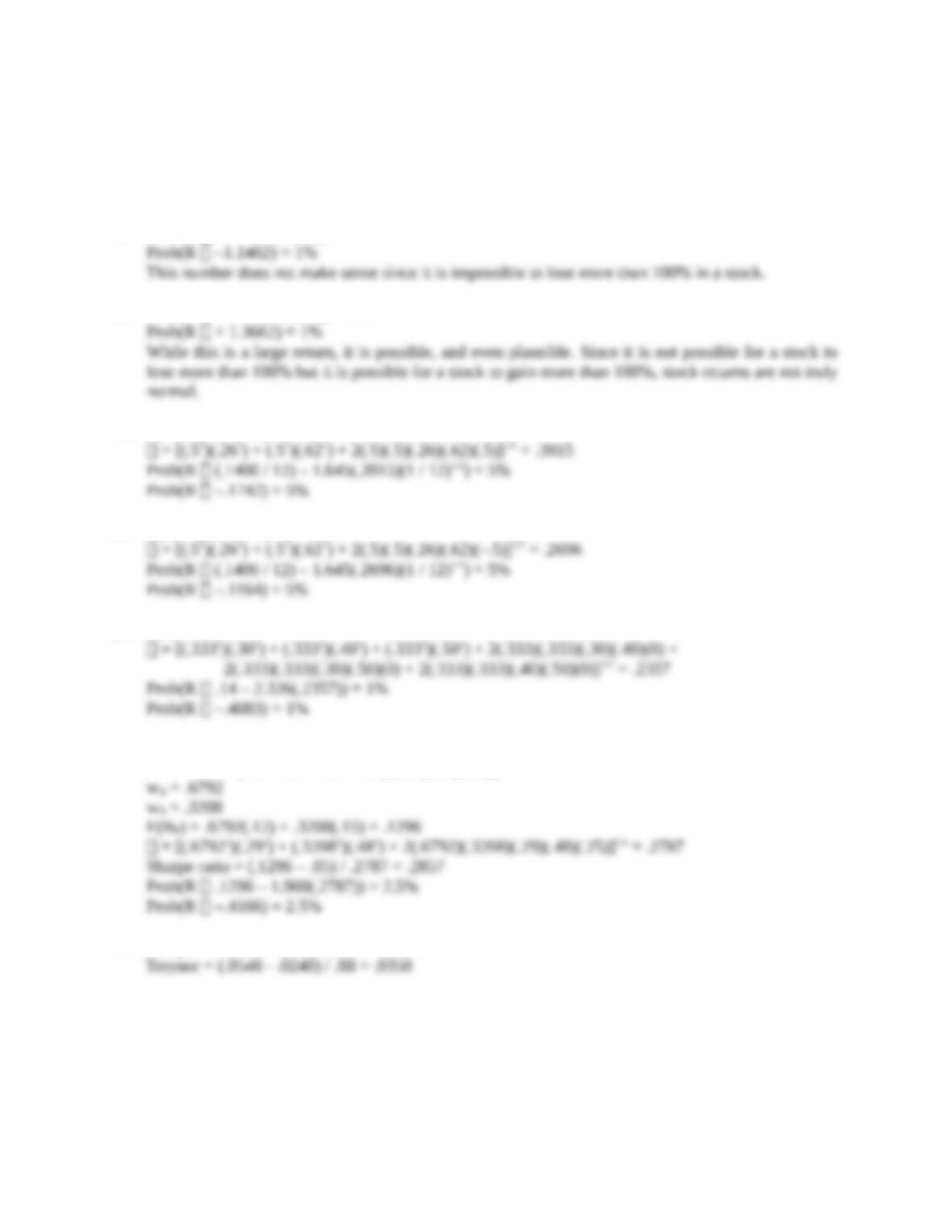12. Any portfolio of the two securities will also have the same expected return.
2σB
2)1/2 =E(RB) - R f
(xS
2σS
2+ xB
2σB
2)1/2
13. Prob(R .11 – 2.326(.54)) = 1%
14. Prob(R .11 + 2.326(.54)) = 1%
15. E(R) = (.10 + .18) / 2 = .1400
16. E(R) = (.10 + .18) / 2 = .1400
17. E(R) = .14
18. wA = [(.12 – .05)(.482) – (.15 – .05)(.29)(.48)(.25)] / {(.12 – .05)(.482) + (.15 – .05)(.292)
– (.12 – .05 + .15 – .05)[(.29)(.48)(.25)]}
19. Sharpe = (.0546 - .0240) / .1505 = .2034
20. First find the average returns of the fund, the market and the risk free rate, which are 5.46%, 1.96%,
Education.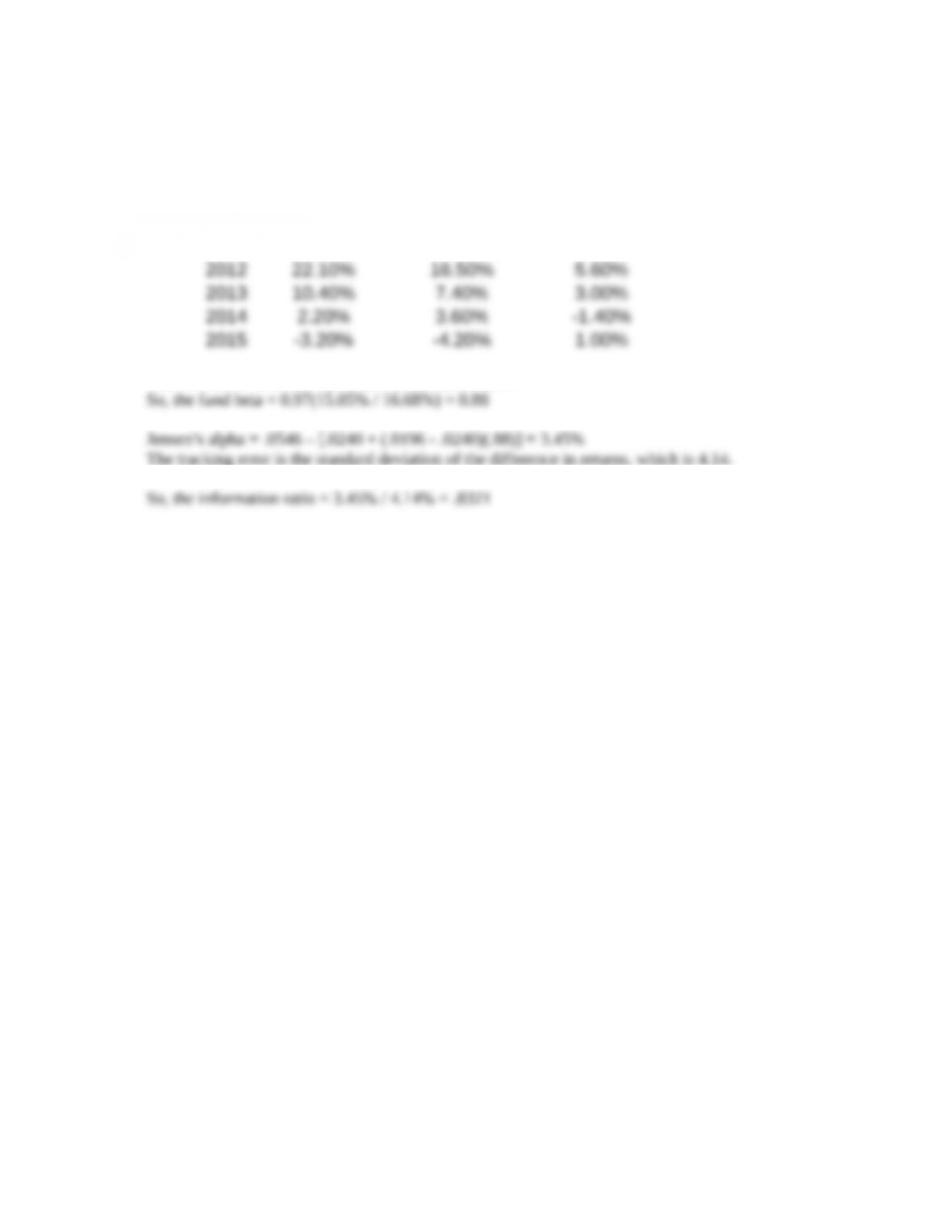2.40%, respectively. The deviations for the fund and the market returns are 15.05% and 16.68%,
respectively.
Next find the excess return over the risk free rate, as well as the difference between the fund and
market returns.
Fund Excess Market Excess Difference
2011 -16.20% -25.50% 9.30%
The correlation between the fund and the market is .97.
The tracking error is the standard deviation of the difference in returns, which is 4.14.
Education.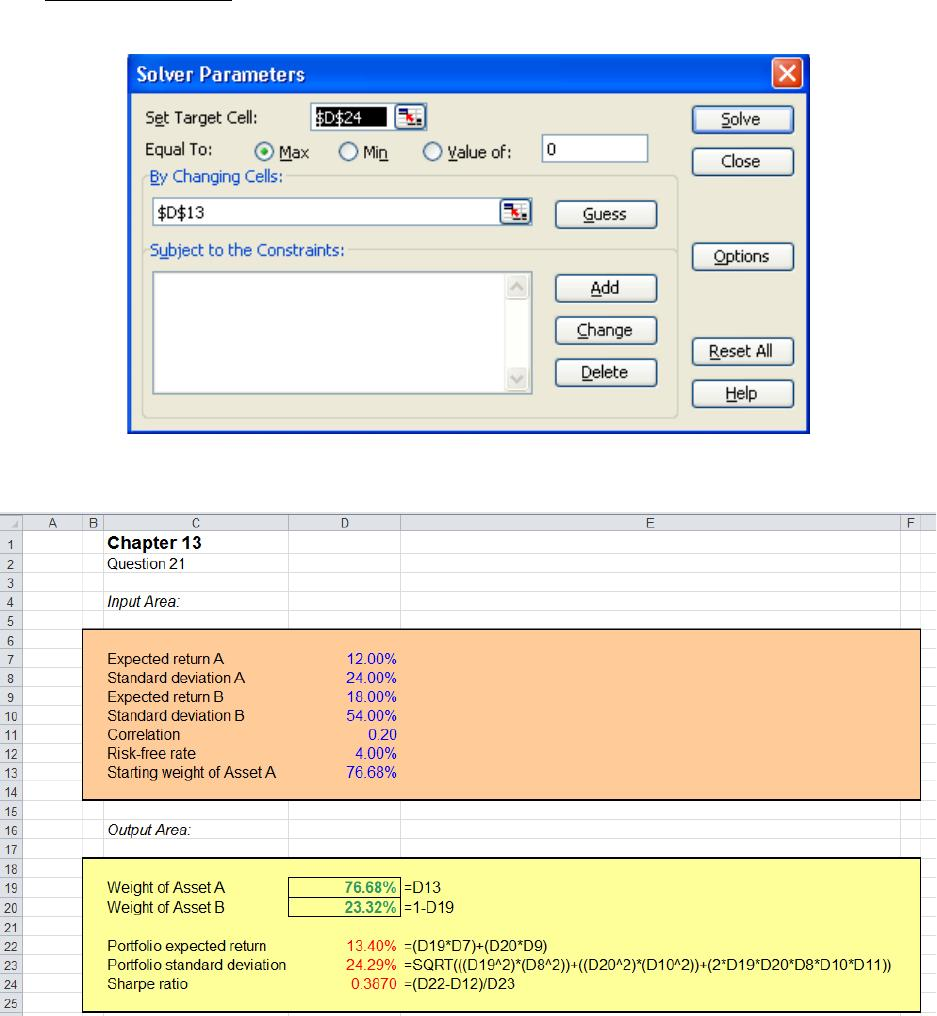21. The Solver inputs are:
Education.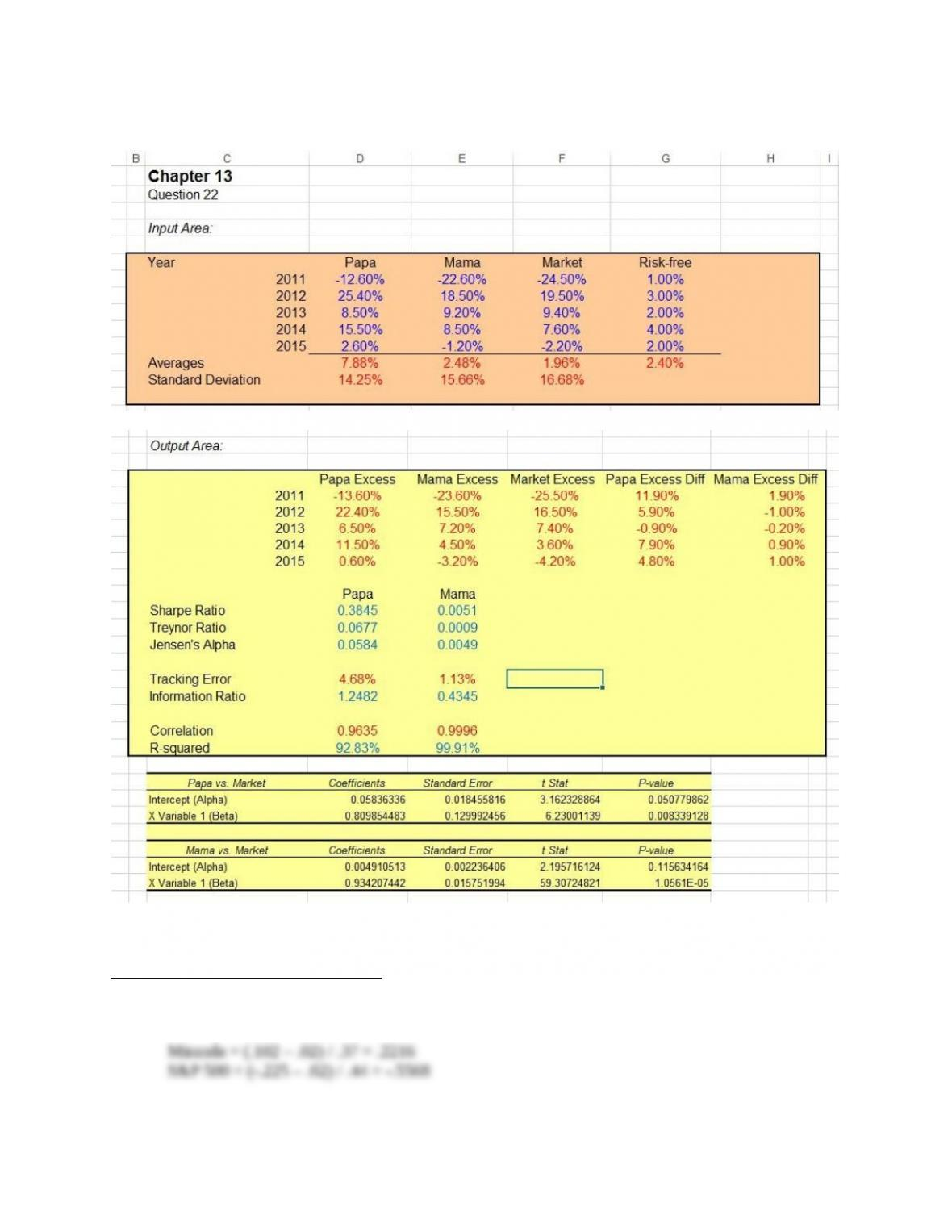22.
CFA Exam Review by Kaplan Schweser
1. a